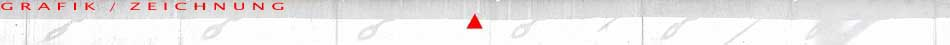M O Z A R T: V E R S U C H E ..Z U.. P A R T I T U R E N G R A F I S C H E   A R B E I T E N U N T E R W E G S E N T W Ü R F E   Z U   B A U S T E L L E N N O T A T I O N E N    (Galerie in Arbeit) S C H U T Z R A U M    A R N O L D S T R A ß E B A C H - C H O R E O G R A F I E N E N T W Ü R F E    Z U M   T A N Z - T H E A T E R A K T